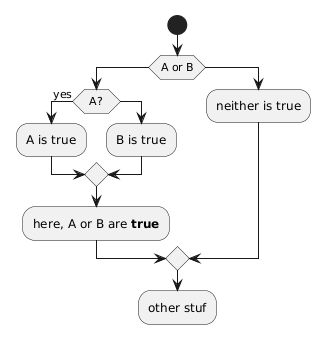# How to flowchart A OR B in activity diagram?

I want to flowchart A OR B in an activitty diagram. Can it even be done? How?

(start)

|

^

/   \  yes

<   A   >-------------->|

\   /                 |

V                   |

|                   |

^                   |

/   \   yes           |

<   B   >-------------->|

\   /                 |

V           +----------------+

|           | a or b is true |

|           +----------------+

|                   |

+---------+

| neither |

| is true |

+---------+

answered Apr 28, 2016 by (15,520 points)

Hello,

start
if (A) then (yes)
else if (B) then (yes)
else
:neither is true;
detach
endif
:A or B;
commented Apr 28, 2016 by anonymous
If there's a way to connect paths afterward, this could work.
answered Apr 28, 2016 by (293,820 points)

Or you can have:

start
if (A or B) then
if (A?) then (yes)
:A is true;
else
:B is true;
endif
:here, A or B are <b>true</b>;
else
:neither is true;
endif
:other stuf;